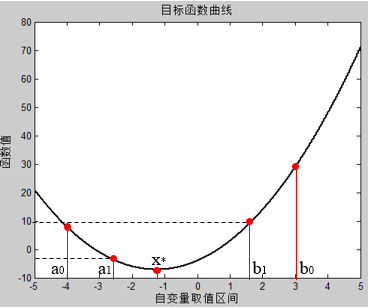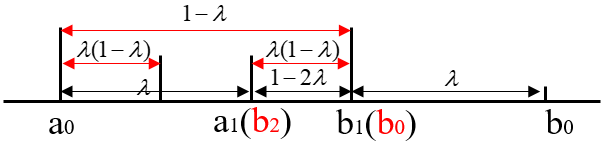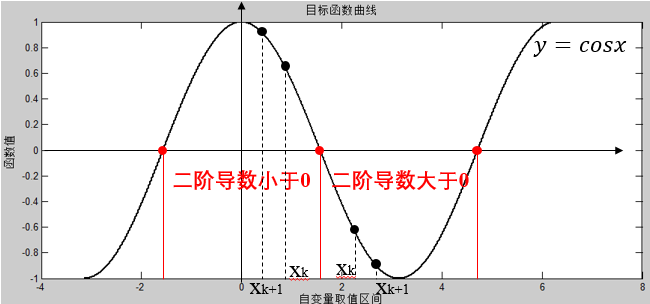深度学习中的优化方法（一）

1. 黄金分割法（只使用目标函数值 $f$
2. 二分法（只使用目标函数的一阶导数 $f'$
3. 牛顿法（使用目标函数的一阶和二阶导数 $f',f''$

一、 黄金分割法

1. 在区间内寻找两个点 $a_{0}, b_{0}$，并使其满足：$a_{0}-a_{1}=b_{0}-b_{1}=\lambda(b_{0}-a_{0}),\lambda<\dfrac{1}{2}$;
2. 计算目标函数在$a_{1}, b_{1}$处的值，如果 $f(a_{1})<f(b_{1})$，则 $x^{*}\in[a_{0},b_{1}]$；如果 $f(a_{1})>f(b_{1})$，则 $x^{*}\in[a_{1},b_{0}]$。如此往复，继续在区间内进行压缩，直到满足误差条件（可以设置为压缩后的区间长度，上一次和下一次之间的差值等）;
3. $f(a_{1})<f(b_{1})$ 为例，此时 $x^{*}\in[a_{0},b_{1}]$，由于 $a_{1}$ 已经在区间内，因此可令 $b_{2}=a_{1}, f(b_{2})=f(a_{1})$，这样下一次迭代时便只需要重新计算 $a_{2}$$f(a_{2})$ 即可。整个过程如下图所示：function [xmin, ymin] = goldensearch( Start, End, e )
%   黄金分割法在闭区间进行一维搜索
%   输入参数：Start代表区间开始位置，End代表区间结束位置，e代表目标区间长度
%   输出参数：xmin表示取得最小值时的坐标，ymin表示求得的最小值
left = Start; right = End; %收敛区间
length = e;     %收敛精度
r = (sqrt(5)-1)/2;  %收敛比例
step = 0;   % 迭代次数初始化
f = f = @(x)2*x^2+5*x-4;;
while right-left>length
step = step+1;
a1 = left+(1-r)*(right-left);
b1 = left+r*(right-left);
ya1 = feval(f, a1);  %计算两端的函数值
yb1 = feval(f, b1);
if ya1 < yb1
right = b1;
b1 = a1;
yb1 = ya1;
a1 = left+r*(right-left);
ya1 = feval(f, a1);
else
left = a1;
b1 = a1;
yb1 = ya1;
a1 = left+(1-r)*(right-left);
ya1 = feval(f, a1);
end
end
%% 输出
xmin = (left+right)/2;
ymin = feval(f, xmin);
fprintf('程序经过%d次迭代的最小值点为%d,最小值为%d\n ',step,xmin,ymin)
%% 绘制图像
x = Start:0.01:End;
y = 2*x^2+5*x-4;
plot(x,y)
hold on
plot(xmin,ymin,'r*') % 在图像中标出极小值点
end

二、二分法

1. 确定区间的中点 $x_{0}=(left+right)/2$
2. 判断目标函数 $f(x)$ 的导数 $f'(x)$$x_{0}$ 处的正负，如果 $f'(x_{0})>0$，那么极小值点 $x^{*}\in [a_{0},x_{0}]$；如果 $f'(x_{0})<0$，那么极小值点 $x^{*}\in [x_{0},b_{0}]$
3. 收缩区间，继续进行迭代求解。

function [xmin, ymin] = binarysearch( Start, End, e )
%   二分法在闭区间进行一维搜索
%   输入参数：Start代表区间开始位置，End代表区间结束位置，e代表目标区间长度
%   输出参数：xmin表示取得最小值时的坐标，ymin表示求得的最小值
left = Start; right = End; %收敛区间
length = e;     %收敛精度
step = 0;   % 迭代次数初始化
f = @(x)cos(x);	% 目标函数
F = @(x)-sin(x); % 目标函数的一阶导数
while right-left>length
step =step+1;
x0=(left+right)/2;
if feval(F, x0) > 0
right=x0;
else
left=x0;
end
end
%% 输出
xmin = (left+right)/2;
ymin = feval(f, xmin);
fprintf('程序经过%d次迭代的最小值点为%d,最小值为%d\n ',step,xmin,ymin)
%% 绘制图像
x = Start:0.01:End;
y = cos(x);
plot(x,y)
hold on
plot(xmin,ymin,'r*') % 在图像中标出极小值点
end

三、牛顿法

$q(x)=f(x^{k})+f'(x^{k})(x-x^{k})+\dfrac{1}{2}f''(x^{k})(x-x^{k})^{2}$

$\dfrac{dq(x)}{dx}|_{x=x^{k+1}}=f'(x^{k})+f''(x^{k})(x^{k+1}-x^{k})=0$function [xmin, ymin] = newton( start, e )
%   牛顿法求解局部最优解
%   输入参数：start代表自变量初始值，e表示x^{k+1}与x^{k}之间的差值
%   输出参数：xmin表示取得最小值时的坐标，ymin表示求得的最小值
length = e;     %收敛精度
step = 0;   % 迭代次数初始化
f = @(x)x^2/2;
F = @(x)x;
while step<=50
step =step+1;
x1 = start-feval(F, start);
f_x0 = feval(f, start); f_x1 = feval(f, x1);
if abs(f_x0-f_x1) <= length
break;
end
start = x1;
x1 = start-feval(F, start);
end
%% 输出
xmin = (start+x1)/2;
ymin = feval(f, xmin);
fprintf('程序经过%d次迭代的最小值点为%d,最小值为%d\n ',step,xmin,ymin)
%% 绘制图像
x = -20:0.0001:20;
y = x.^2/2;
plot(x,y)
hold on
plot(xmin,ymin,'r*') % 在图像中标出极小值点
end
posted @ 2021-07-27 17:38  ZhiboZhao  阅读(276)  评论(0编辑  收藏  举报